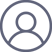李兴旺[转载]Neyman–Pearson lemma
2018-11-2 20:35

In statistics, the Neyman–Pearson lemma was introduced by Jerzy Neyman and Egon Pearson in a paper in 1933.

Suppose one is performing a hypothesis test between two simple hypotheses H0θ = θ0 and H1θ = θ1 using the likelihood-ratio test with threshold, which rejects H0 in favour of H1 at a significance level of

•where

•andis the likelihood function. Then, the lemma states thatis the most powerful test at significance level α.

If the test is most powerful for all, it is said to be uniformly most powerful (UMP) for alternatives in the set.

In practice, the likelihood ratio is often used directly to construct tests — see likelihood-ratio test. However it can also be used to suggest particular test-statistics that might be of interest or to suggest simplified tests — for this, one considers algebraic manipulation of the ratio to see if there are key statistics in it related to the size of the ratio (i.e. whether a large statistic corresponds to a small ratio or to a large one).

## Proof

Define the rejection region of the null hypothesis for the Neyman–Pearson (NP) test as

•whereis chosen so thatAny other test will have a different rejection region that we denote by. The probability of the data falling in regiongiven parameteris

•For the test with critical regionto have level, it must be true that, hence

•It will be useful to break these down into integrals over distinct regions:

•Setting, these two expressions and the above inequality yield that

•The powers of the two tests areand, and we would like to prove that:

•However, as shown above this is equivalent to:in what follows we show that the above inequality holds:

•## Example

Letbe a random sample from thedistribution where the meanis known, and suppose that we wish to test foragainst. The likelihood for this set of normally distributed data is

•We can compute the likelihood ratio to find the key statistic in this test and its effect on the test's outcome:

•This ratio only depends on the data through. Therefore, by the Neyman–Pearson lemma, the most powerful test of this type of hypothesis for this data will depend only on. Also, by inspection, we can see that if, thenis a decreasing function of. So we should rejectifis sufficiently large. The rejection threshold depends on the size of the test. In this example, the test statistic can be shown to be a scaled Chi-square distributed random variable and an exact critical value can be obtained.

## Application in economics

A variant of the Neyman–Pearson lemma has found an application in the seemingly unrelated domain of the economics of land value. One of the fundamental problems in consumer theory is calculating the demand function of the consumer given the prices. In particular, given a heterogeneous land-estate, a price measure over the land, and a subjective utility measure over the land, the consumer's problem is to calculate the best land parcel that he can buy – i.e. the land parcel with the largest utility, whose price is at most his budget. It turns out that this problem is very similar to the problem of finding the most powerful statistical test, and so the Neyman–Pearson lemma can be used.

## Uses in electronics engineering

The Neyman–Pearson lemma is quite useful in electronics engineering, namely in the design and use of radar systems, digital communication systems, and in signal processing systems. In radar systems, the Neyman–Pearson lemma is used in first setting the rate of missed detections to a desired (low) level, and then minimizing the rate of false alarms, or vice versa. Neither false alarms nor missed detections can be set at arbitrarily low rates, including zero. All of the above goes also for many systems in signal processing.

## Uses in particle physics

The Neyman–Pearson lemma is applied to the construction of analysis-specific likelihood-ratios, used to e.g. test for signatures of new physics against the nominal Standard Model prediction in proton-proton collision datasets collected at the LHC.

## References

1. Jump up^ Neyman, J.; Pearson, E. S. (1933-02-16). "IX. On the problem of the most efficient tests of statistical hypotheses"Phil. Trans. R. Soc. Lond. A231 (694–706): 289–337. doi:10.1098/rsta.1933.0009ISSN 0264-3952.

2. Jump up^ Berliant, M. (1984). "A characterization of the demand for land". Journal of Economic Theory33 (2): 289. doi:10.1016/0022-0531(84)90091-7.

• E. L. Lehmann, Joseph P. Romano, Testing statistical hypotheses, Springer, 2008, p. 60